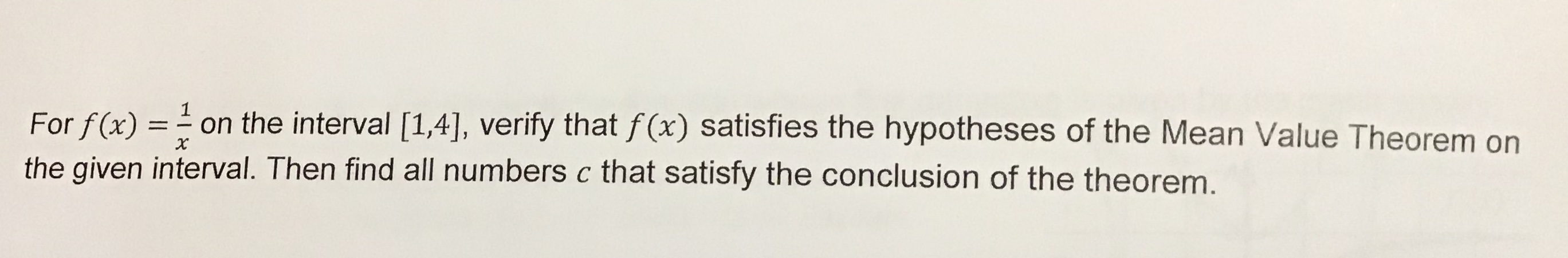# 1on the interval [1,4], verify that f(x) satisfies the hypotheses of the Mean Value Theorem onFor f(x)the given interval. Then find all numbers c that satisfy the conclusion of the theorem.

Question
2 viewshelp_outlineImage Transcriptionclose1 on the interval [1,4], verify that f(x) satisfies the hypotheses of the Mean Value Theorem on For f(x) the given interval. Then find all numbers c that satisfy the conclusion of the theorem. fullscreen
check_circle

Step 1

To verify the hypotheses of the mean value theorem and find all numbers c that satisfy the conclusion of the theorem.

Step 2

Given information:

Step 3

Hypotheses of Mean value theorem:

(1). Function f is continuous on the closed interval [a, b].

(2). Function f is diffe...

### Want to see the full answer?

See Solution

#### Want to see this answer and more?

Solutions are written by subject experts who are available 24/7. Questions are typically answered within 1 hour.*

See Solution
*Response times may vary by subject and question.
Tagged in

### Continuity